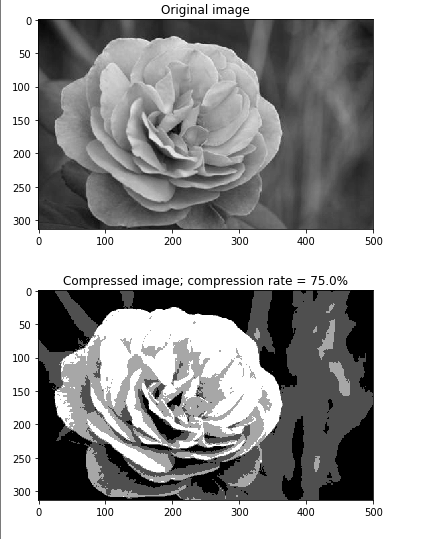# python实现使用聚类算法进行图片压缩

## python实现使用聚类算法进行图片压缩

import numpy as np
from scipy import misc
from sklearn import cluster
import matplotlib.pyplot as plt

###STEP1###
#compress_image函数实现图片压缩功能，compress_image函数将每个像素作为一个元素进行聚类，以此减少其颜色个数。
#参数img是图片传入的接口，因此我们需要知道变量img的数据结构，请自行查看。
def compress_image(img, num_clusters):
#思考，聚类算法对输入的数据结构要求如何？
#问题一：补全代码，将img结构进行转换即每个像素作为一个元素，使之能符合聚类算法数据输入的要求。
X = img.reshape((-1, 1))    #将原来的img文件的数据结构转化为单列格式。不知道img原来的数据结构可能是一个矩阵，我们将每一个像素点都转化为单列的格式。

#print(X.shape)
# 创建KMeans聚类模型，并训练。
kmeans = cluster.KMeans(n_clusters=num_clusters, n_init=4, random_state=5)
kmeans.fit(X)

#问题二：补全代码，分别获取每个数据聚类后的label，以及每个label的质心。
centroids = kmeans.cluster_centers_.squeeze()  #把img文件新生成的数组将其数组形状中的维度删除 也就是将shape中为1的删除。
labels = kmeans.labels_                        #那到新数据的质心，目的要替换原来像素点的质心

#问题三：使用质心的数值代替原数据的label值，那么我们将获得一个新的图像。
# 提示，使用numpy的choose函数进行进行质心值的代替，reshape函数回复原图片的数据结构，并返回结果。
input_image_compressed = np.choose(labels, centroids).reshape(img.shape)
#使用choose函数将质心替换原来的数据。

return input_image_compressed

###STEP2###
#plot_image函数打印图片
def plot_image(img, title):
vmin = img.min()
vmax = img.max()
plt.figure()
plt.title(title)
plt.imshow(img, cmap=plt.cm.gray, vmin=vmin, vmax=vmax)

###STEP3###
#读入图片，设置压缩率，实现压缩
if __name__=='__main__':
#设置图片的路径和压缩比例
input_file = "E:/flower/flower.jpg"
num_bits = 2
if not 1 <= num_bits <= 8:
raise TypeError('Number of bits should be between 1 and 8')
num_clusters = np.power(2, num_bits)
# 输出压缩的比例
compression_rate = round(100 * (8.0 - num_bits) / 8.0, 2)
print ("\nThe size of the image will be reduced by a factor of", 8.0/num_bits)
print ("\nCompression rate = " + str(compression_rate) + "%")
# 加载需要压缩的图片
# 原始图像的输出
plot_image(input_image, 'Original image')
# 压缩后的图像输出
input_image_compressed = compress_image(input_image, num_clusters)
plot_image(input_image_compressed, 'Compressed image; compression rate = '
+ str(compression_rate) + '%')

plt.show()©️2019 CSDN 皮肤主题: 大白 设计师: CSDN官方博客